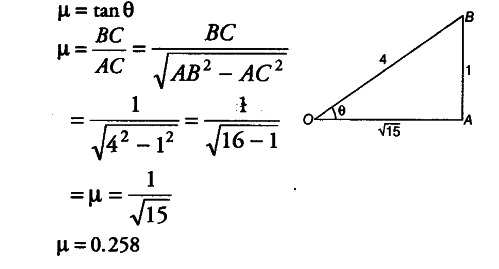# A body placed on a rough inclined plane just begins to slide

A body placed on a rough inclined plane just begins to slide, when the slope of the plane equal to 1 in 4. Calculate the coefficient of friction.

The slope of the plane equal to 1 in 4 implies that if BC = 1 and AB = 4. Suppose that the plane is inclined at angle \theta with the horizontal AC. From the relation between the coefficient of friction and angle of repose, we have